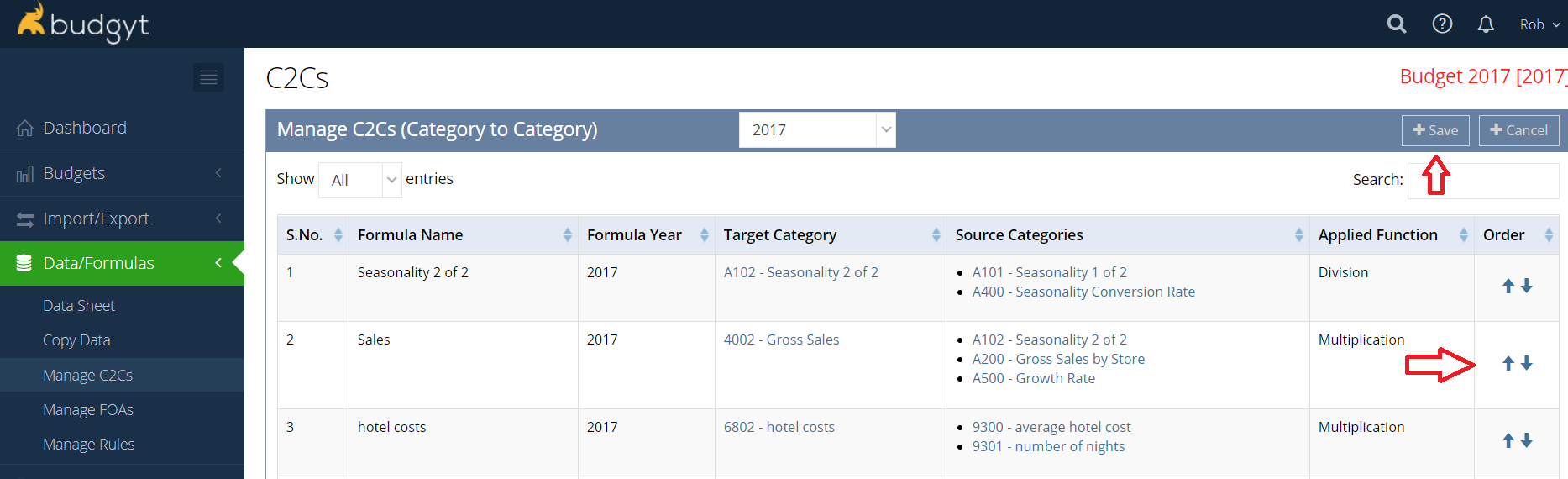Community Knowledge Base

# My C2C/FOA formulas are not calculating correctly.

Formulas in Budgyt are executed in order. If it seems that some of your formulas are not calculating as expected, they may be in the wrong order.

For instance, if you use the result of Formula A as one of the sources for Formula B, then Formula A must be calculated before Formula B to provide the right result to B

To check the order of your formulas, go to Data/Formulas > Manage C2C/FOA. The formulas are calculated in the order that they appear on the screen, from top to bottom.

Budgyt will check the order for you, and flag any that are out of order, but it is possible to ignore the error message. If there are any out of order, you will still see the error message when you return to the Manage C2Cs screen.

To fix the ordering, click Reorder at the top right of your screen, and use the arrows on the right, or drag and drop the formula to the correct location.

Also, it is important to note that Budgyt calculates ALL C2C formulas BEFORE it works on the FOAs. This means that you cannot build a C2C if one of its source categories is the result of an FOA calculation.